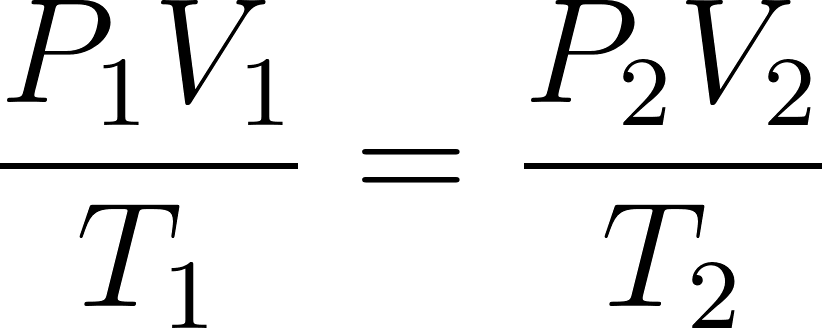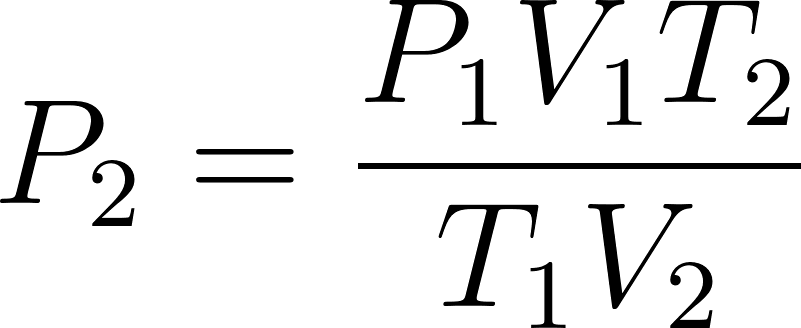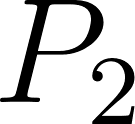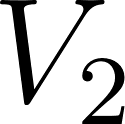×
Get Full Access to Introductory Chemistry - 5 Edition - Chapter 11 - Problem 53p
Get Full Access to Introductory Chemistry - 5 Edition - Chapter 11 - Problem 53p

×

# Solved: A scuba diver takes a 2.8-L balloon from the surface, where the pressure is 1.0ISBN: 9780321910295 34

## Solution for problem 53P Chapter 11

Introductory Chemistry | 5th Edition

• Textbook Solutions
• 2901 Step-by-step solutions solved by professors and subject experts
• Get 24/7 help from StudySoup virtual teaching assistantsIntroductory Chemistry | 5th Edition

4 5 1 399 Reviews
30
0
Problem 53P

PROBLEM 53P

A scuba diver takes a 2.8-L balloon from the surface, where the pressure is 1.0 atm and the temperature is 34 °C, to a depth of 25 m, where the pressure is 3.5 atm and the temperature is 18 °C. What is the volume of the balloon at this depth?

Step-by-Step Solution:

Problem 53P

A scuba diver takes a 2.8-L balloon from the surface, where the pressure is 1.0 atm and the temperature is 34 °C, to a depth of 25 m, where the pressure is 3.5 atm and the temperature is 18 °C. What is the volume of the balloon at this depth?

Step by step solution

Step 1 of 2

According to the combined gas law,.....................(1)

Rearrange the equation (1)............................(2)

Here,= Initial pressure of the gas= Initial volume of the gas= Initial temperature= final pressure of the gas= final volume of the gas= final temperature

Step 2 of 2

##### ISBN: 9780321910295

Unlock Textbook Solution